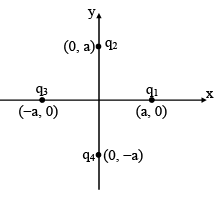Four charges q1, q2, q3 and q4 are placed at ...
Four charges q1, q2, q3 and q4 are placed at the positions as shown in the figure, given q1 +q2+ q3 + q4 = 0 . The electric field on z-axis• a)
is always along +ve z-axis
• b)
is always along −ve z-axis
• c)
is always zero on z-axis
• d)
may be perpendicular to z-axis or zero on z-axis depending on charges
Correct answer is option 'D'. Can you explain this answer?

### Related TestSatvir Kaur Jul 22, 2020Related Four charges q1, q2, q3 and q4 are placed at the positions as shown in the figure, given q1 +q2+ q3 + q4 = 0 . The electric field on z-axis a)is always along +ve z-axis b)is always along ve z-axis c)is always zero on z-axis d)may be perpendicular to z-axis or zero onz-axis depending on chargesCorrect answer is option 'D'. Can you explain this answer?
As q1 +q2 + q3 + q4 = 0 , z axis is an equipotential line. Thus electric field is either zero or perpendicular to the z-axis. When q2 = q4 and q3 = q1 electric field is zero at origin and in all other cases it is perpendicular to z-axis.

 1 Crore+ students have signed up on EduRev. Have you?